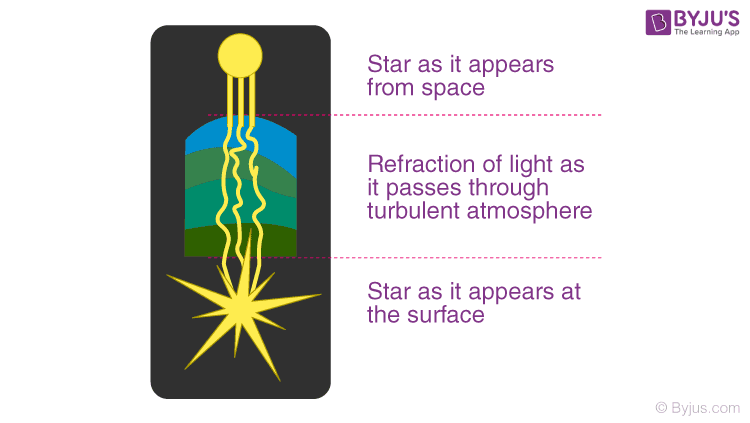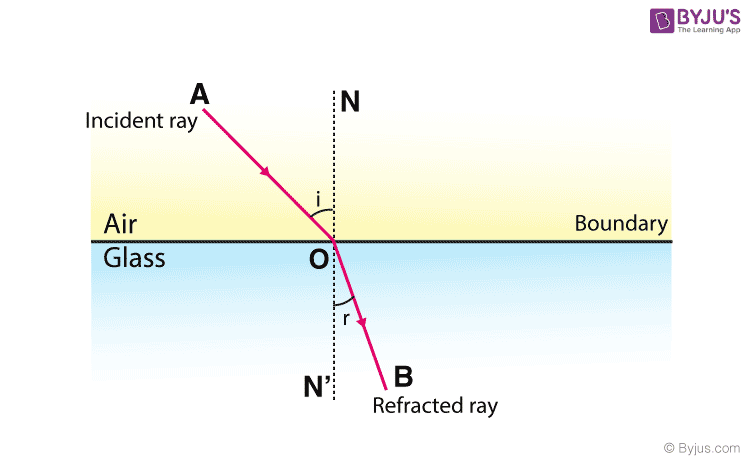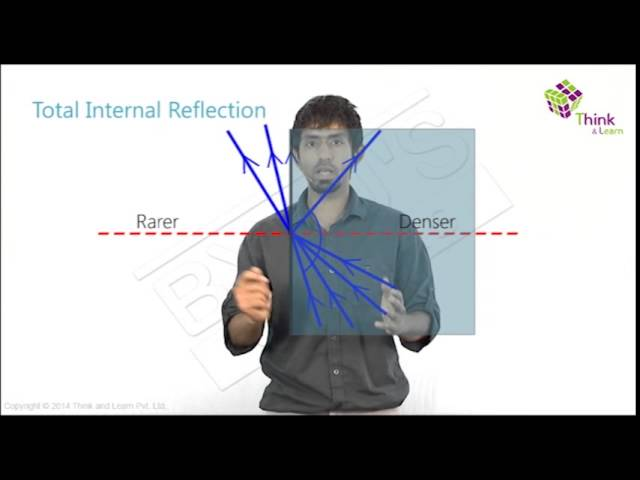# Refraction of Light

## Refraction Explanation

Refraction is the bending of a wave when it passes from one medium to another. The bending is caused due to the differences in density between the two substances.

### Defining Refraction

“Refraction is the change in the direction of a wave passing from one medium to another.”

Refraction of light is one of the most commonly observed phenomena, but other waves like sound waves and water waves also experience refraction. Refraction makes it possible for us to have optical instruments such as magnifying glasses, lenses and prisms. It is also because of the refraction of light that we are able to focus light on to our retina.

Refraction in Nature#### Why do stars twinkle?

Did you know that the twinkling effect of stars is due to atmospheric refraction? The starlight undergoes several refractions while reaching the Earth. This atmospheric refraction occurs in a medium of gradually changing refractive index.

## Causes of Refraction

### Change of Speed Results in Change in Direction

A light ray refracts whenever it travels at an angle into a medium of different refractive index. This change in speed results in a change in direction. As an example, consider air travelling into water. The speed of light decreases as it continues to travel at a different angle.Refraction of light in glass is shown in the figure above. When light travels from air into glass, the light slows down and changes direction slightly. When light travels from a less dense substance to a denser substance, the refracted light bends more towards the normal line. If the light wave approaches the boundary in a direction that is perpendicular to it, the light ray doesn’t refract in spite of the change in speed.

## Laws of Refraction of Light

Laws of refraction state that:

• The incident ray refracted ray, and the normal to the interface of two media at the point of incidence all lie on the same plane.
• The ratio of the sine of the angle of incidence to the sine of the angle of refraction is a constant. This is also known as Snell’s law of refraction.
 $\frac{sin\;i}{sin\;r}=constant$

Watch the video below to understand the process of refraction in detail## What is Refractive Index?

Refractive index, also called the index of refraction describes how fast light travels through the material.

Refractive Index is dimensionless. For a given material, the refractive index is the ratio between the speed of light in a vacuum (c) and the speed of light in the medium (v). If the refractive index for a medium is represented by n, then it is given by the following formula:

 $~~~~~~~$ $n = \frac {c}{v}$

Based on the refractive index of the medium, the light ray changes its direction, or it bends at the junction separating the two media. If the light ray travels from a medium to another of a higher refractive index, it bends towards the normal, else it bends away from the normal.

## Refraction of Light in Real Life

• Mirage and looming are optical illusions which are a result of refraction of light.
• A swimming pool always looks shallower than it really is because the light coming from the bottom of the pool bends at the surface due to refraction of light.
• Formation of a rainbow is an example of refraction as the sun rays bend through the raindrops resulting in the rainbow.
• When white light passes through a prism it is split into its component colours – red, orange, yellow, green, blue and violet due to refraction of light.

## Applications of Refraction of Light

Refraction has many applications in optics and technology. A few of the prominent applications are listed below:

• A lens uses refraction to form an image of an object for various purposes, such as magnification.
• Spectacles worn by people with defective vision use the principle of refraction.
• Refraction is used in peepholes of house doors, cameras, movie projectors and telescopes.

## Refraction Solved Problems

1. Light travelling in air enters into an optical fibre of refractive index 1.44.
a) In which direction does the light bend?
b) If the angle of incidence on one end of the fibre is 22o, then what is the angle of refraction?

Solution:
a) The light travels from rarer medium(air) to denser medium(optical fibre), hence the refracted ray will bend towards the normal.
b) The angle of refraction can be calculated as follows:
Let air be medium 1 and optical fibre be medium 2. Therefore, n1 = 1.00, n2 = 1.44, and θ1= 22o.
Now, substituting the values in the equation as follows:
(1.00) sin 22o = 1.44 sin θ2.
sin θ2 = (1.00/1.44) sin 22o = 0.260
θ2 = sin-1 (0.260) = 15o

2. The light travelling through the optical fibre reaches the end of the optical fibre and exits into the air. If the angle of incidence at the end of the tube is 30o. Then what would the angle of refraction outside the fibre be?

Solution:
Let the fibre be medium 1 and air medium 2. Therefore, n1 = 1.44, n2 = 1.00, and θ,1 = 30o. Substituting the values in the equation, we get
(1.44) sin 30o = 1.00 sin θ2
sin θ2 = (1.44/1.00) sin 30o = 1.44 (0.500) = 0.720
θ2 = sin-1 (0.720) = 46o
This time we notice that the angle of refraction is larger than the angle of incidence. This indicates that the light is bending away from the normal as it enters a rarer material.## Frequently Asked Questions – FAQs

### Define Refraction.

The change in the direction of a wave when it passes from one medium to another is known as refraction.

### When does refraction of waves occur?

The refraction of light occurs when a light wave, incident at an angle away from the normal, passes a boundary from one medium into another in which there is a change in velocity of the light.

### When is the refraction of light not possible?

When the incident light is perpendicular to the boundary, refraction of light is not possible.

### What is the difference between reflection and refraction in the light?

Reflection of light is when the light bounces off a medium. If the medium has a smooth surface, then the angle of incidence is equal to the angle of reflection. Refraction of light is the change in the direction of light as it passes from one medium to another.

### State an example of refraction of light.

An example of refraction is rainbow. The light rays bend as they enter water drops in the atmosphere forming a rainbow.

Stay tuned to BYJU’S and Fall in Love with Learning!

1. Arijit Bhakta

2. Jenmini yekar

It’s kinda good🖤👍👍👌👌

3. Prasahya

This explanation of Refraction of light gave me a very good understanding.

This is very good platform for students to get good marks in exams 🙌 this helps mi so much in may doubts so thank you so much

1. shital kamble

BYJU’S is a good app that enables students to score good marks in exams.

2. KARAN SHMBI

YES YOU ARE RIGHT

5. Tenzin pema

The above notes made my work of making rough note really easy. Thank you so much and it is really easy to understand and very useful.
THANK U SO MUCH!!!!!

6. Bhawya Srivastav

Yeah!! This was really helpful for my physics project work😊😊

7. KARAN SEHMBI

OHYEE I GOT A MASSIVE EXPLANATION. I THINK I COULD SCORE 50 ON 50 ON MY TEST.

8. Robin Edwin